Anúncio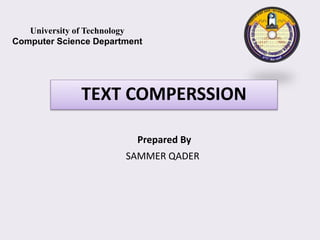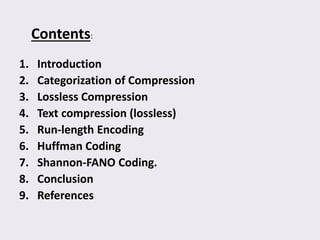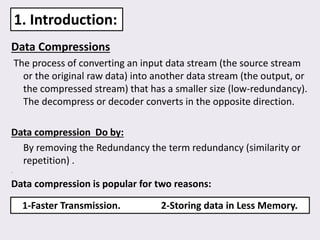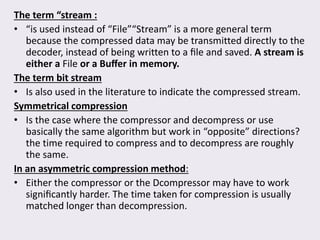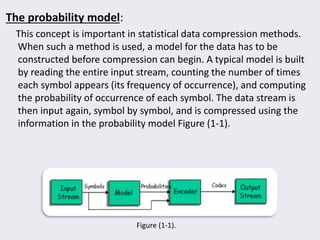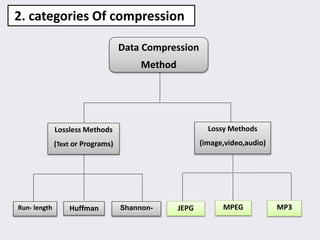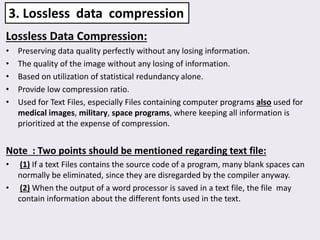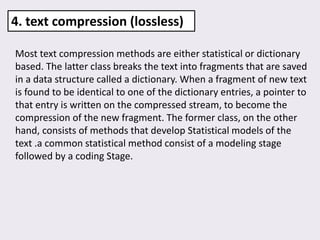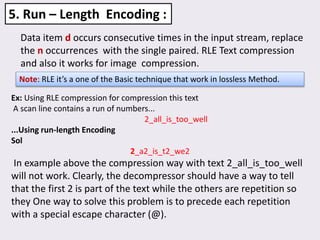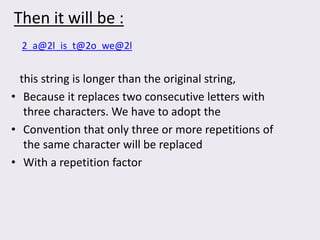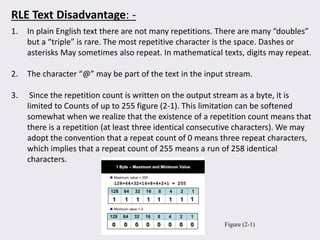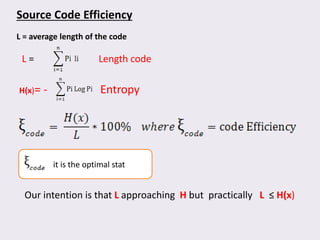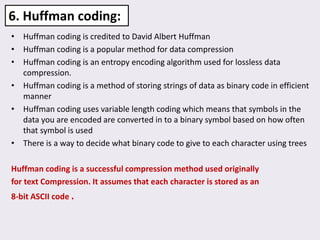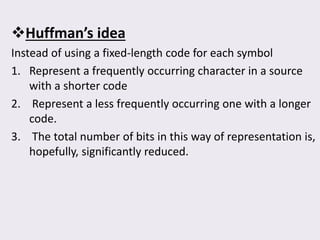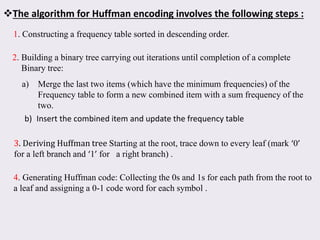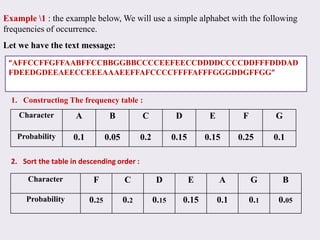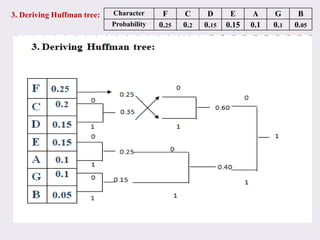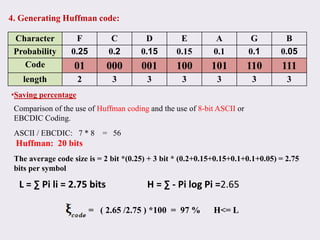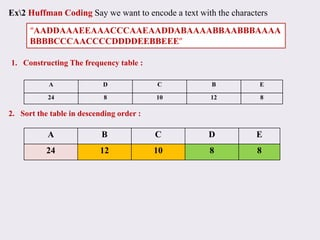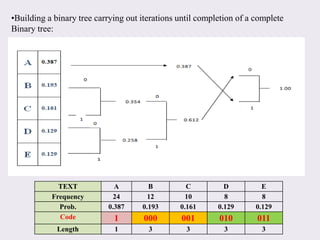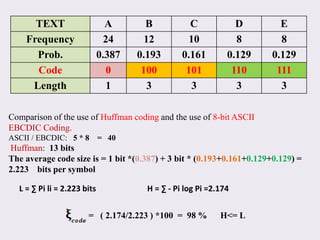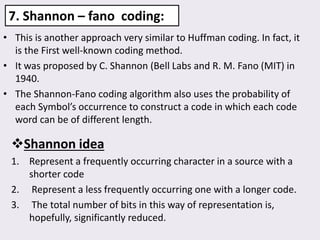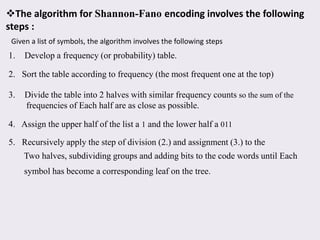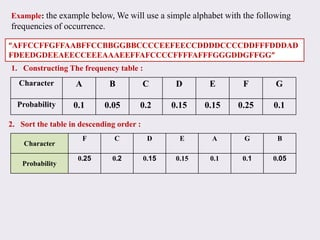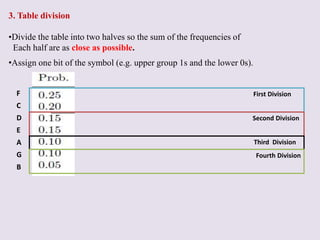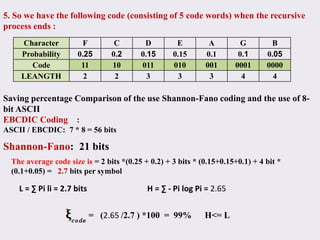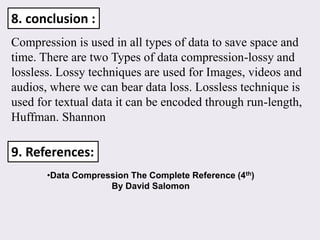1 de 28
Anúncio

### Text compression

1. Prepared By SAMMER QADER TEXT COMPERSSION University of Technology Computer Science Department
2. Contents: 1. Introduction 2. Categorization of Compression 3. Lossless Compression 4. Text compression (lossless) 5. Run-length Encoding 6. Huffman Coding 7. Shannon-FANO Coding. 8. Conclusion 9. References
3. Data Compressions The process of converting an input data stream (the source stream or the original raw data) into another data stream (the output, or the compressed stream) that has a smaller size (low-redundancy). The decompress or decoder converts in the opposite direction. Data compression Do by: By removing the Redundancy the term redundancy (similarity or repetition) . . 1. Introduction: 1-Faster Transmission. 2-Storing data in Less Memory. Data compression is popular for two reasons:
4. The term “stream : • “is used instead of “File”“Stream” is a more general term because the compressed data may be transmitted directly to the decoder, instead of being written to a ﬁle and saved. A stream is either a File or a Buﬀer in memory. The term bit stream • Is also used in the literature to indicate the compressed stream. Symmetrical compression • Is the case where the compressor and decompress or use basically the same algorithm but work in “opposite” directions? the time required to compress and to decompress are roughly the same. In an asymmetric compression method: • Either the compressor or the Dcompressor may have to work signiﬁcantly harder. The time taken for compression is usually matched longer than decompression.
5. The probability model: This concept is important in statistical data compression methods. When such a method is used, a model for the data has to be constructed before compression can begin. A typical model is built by reading the entire input stream, counting the number of times each symbol appears (its frequency of occurrence), and computing the probability of occurrence of each symbol. The data stream is then input again, symbol by symbol, and is compressed using the information in the probability model Figure (1-1). Figure (1-1).
6. 2. categories Of compression Data Compression Method Lossy Methods (image,video,audio) Lossless Methods (Text or Programs) HuffmanRun- length Huffman Shannon- JEPG MPEG MP3
7. Lossless Data Compression: • Preserving data quality perfectly without any losing information. • The quality of the image without any losing of information. • Based on utilization of statistical redundancy alone. • Provide low compression ratio. • Used for Text Files, especially Files containing computer programs also used for medical images, military, space programs, where keeping all information is prioritized at the expense of compression. Note : Two points should be mentioned regarding text file: • (1) If a text Files contains the source code of a program, many blank spaces can normally be eliminated, since they are disregarded by the compiler anyway. • (2) When the output of a word processor is saved in a text file, the file may contain information about the different fonts used in the text. 3. Lossless data compression
8. Most text compression methods are either statistical or dictionary based. The latter class breaks the text into fragments that are saved in a data structure called a dictionary. When a fragment of new text is found to be identical to one of the dictionary entries, a pointer to that entry is written on the compressed stream, to become the compression of the new fragment. The former class, on the other hand, consists of methods that develop Statistical models of the text .a common statistical method consist of a modeling stage followed by a coding Stage. 4. text compression (lossless)
9. Data item d occurs consecutive times in the input stream, replace the n occurrences with the single paired. RLE Text compression and also it works for image compression. 5. Run – Length Encoding : Note: RLE it’s a one of the Basic technique that work in lossless Method. Ex: Using RLE compression for compression this text A scan line contains a run of numbers... 2_all_is_too_well ...Using run-length Encoding Sol 2_a2_is_t2_we2 In example above the compression way with text 2_all_is_too_well will not work. Clearly, the decompressor should have a way to tell that the first 2 is part of the text while the others are repetition so they One way to solve this problem is to precede each repetition with a special escape character (@).
10. Then it will be : this string is longer than the original string, • Because it replaces two consecutive letters with three characters. We have to adopt the • Convention that only three or more repetitions of the same character will be replaced • With a repetition factor 2_a@2l_is_t@2o_we@2l
11. 1. In plain English text there are not many repetitions. There are many “doubles” but a “triple” is rare. The most repetitive character is the space. Dashes or asterisks May sometimes also repeat. In mathematical texts, digits may repeat. 2. The character “@” may be part of the text in the input stream. 3. Since the repetition count is written on the output stream as a byte, it is limited to Counts of up to 255 figure (2-1). This limitation can be softened somewhat when we realize that the existence of a repetition count means that there is a repetition (at least three identical consecutive characters). We may adopt the convention that a repeat count of 0 means three repeat characters, which implies that a repeat count of 255 means a run of 258 identical characters. RLE Text Disadvantage: - Figure (2-1)
12. Source Code Efficiency L = average length of the code L = Length code H(x)= - Entropy it is the optimal stat Our intention is that L approaching H but practically L ≥ H(x)
13. • Huffman coding is credited to David Albert Huffman • Huffman coding is a popular method for data compression • Huffman coding is an entropy encoding algorithm used for lossless data compression. • Huffman coding is a method of storing strings of data as binary code in efficient manner • Huffman coding uses variable length coding which means that symbols in the data you are encoded are converted in to a binary symbol based on how often that symbol is used • There is a way to decide what binary code to give to each character using trees Huffman coding is a successful compression method used originally for text Compression. It assumes that each character is stored as an 8-bit ASCII code . 6. Huffman coding:
14. Huffman’s idea Instead of using a fixed-length code for each symbol 1. Represent a frequently occurring character in a source with a shorter code 2. Represent a less frequently occurring one with a longer code. 3. The total number of bits in this way of representation is, hopefully, significantly reduced.
15. The algorithm for Huffman encoding involves the following steps : 1. Constructing a frequency table sorted in descending order. 2. Building a binary tree carrying out iterations until completion of a complete Binary tree: a) Merge the last two items (which have the minimum frequencies) of the Frequency table to form a new combined item with a sum frequency of the two. b) Insert the combined item and update the frequency table 3. Deriving Huffman tree Starting at the root, trace down to every leaf (mark ‘0’ for a left branch and ‘1’ for a right branch) . 4. Generating Huffman code: Collecting the 0s and 1s for each path from the root to a leaf and assigning a 0-1 code word for each symbol .
16. Character A B C D E F G Probability 0.1 0.05 0.2 0.15 0.15 0.25 0.1 Example 1 : the example below, We will use a simple alphabet with the following frequencies of occurrence. Let we have the text message: “AFFCCFFGFFAABFFCCBBGGBBCCCCEEFEECCDDDDCCCCDDFFFDDDAD FDEEDGDEEAEECCEEEAAAEEFFAFCCCCFFFFAFFFGGGDDGFFGG” 1. Constructing The frequency table : 2. Sort the table in descending order : Character F C D E A G B Probability 0.25 0.2 0.15 0.15 0.1 0.1 0.05
17. 3. Deriving Huffman tree: Character F C D E A G B Probability 0.25 0.2 0.15 0.15 0.1 0.1 0.05
18. 4. Generating Huffman code: Character F C D E A G B Probability 0.25 0.2 0.15 0.15 0.1 0.1 0.05 Code 01 000 001 100 101 110 111 length 2 3 3 3 3 3 3 Comparison of the use of Huffman coding and the use of 8-bit ASCII or EBCDIC Coding. ASCII / EBCDIC: 7 * 8 = 56 Huffman: 20 bits The average code size is = 2 bit *(0.25) + 3 bit * (0.2+0.15+0.15+0.1+0.1+0.05) = 2.75 bits per symbol •Saving percentage L = ∑ Pi li = 2.75 bits H = ∑ - Pi log Pi =2.65 = ( 2.65 /2.75 ) *100 = 97 % H<= L
19. A D C B E 24 8 10 12 8 A B C D E 24 12 10 8 8 Ex2 Huffman Coding Say we want to encode a text with the characters “AADDAAAEEAAACCCAAEAADDABAAAABBAABBBAAAA BBBBCCCAACCCCDDDDEEBBEEE” 1. Constructing The frequency table : 2. Sort the table in descending order :
20. •Building a binary tree carrying out iterations until completion of a complete Binary tree: TEXT A B C D E Frequency 24 12 10 8 8 Prob. 0.387 0.193 0.161 0.129 0.129 Code 1 000 001 010 011 Length 1 3 3 3 3
21. Comparison of the use of Huffman coding and the use of 8-bit ASCII EBCDIC Coding. ASCII / EBCDIC: 5 * 8 = 40 Huffman: 13 bits The average code size is = 1 bit *(0.387) + 3 bit * (0.193+0.161+0.129+0.129) = 2.223 bits per symbol TEXT A B C D E Frequency 24 12 10 8 8 Prob. 0.387 0.193 0.161 0.129 0.129 Code 0 100 101 110 111 Length 1 3 3 3 3 L = ∑ Pi li = 2.223 bits H = ∑ - Pi log Pi =2.174 = ( 2.174/2.223 ) *100 = 98 % H<= L
22. 7. Shannon – fano coding: • This is another approach very similar to Huffman coding. In fact, it is the First well-known coding method. • It was proposed by C. Shannon (Bell Labs and R. M. Fano (MIT) in 1940. • The Shannon-Fano coding algorithm also uses the probability of each Symbol’s occurrence to construct a code in which each code word can be of different length. Shannon idea 1. Represent a frequently occurring character in a source with a shorter code 2. Represent a less frequently occurring one with a longer code. 3. The total number of bits in this way of representation is, hopefully, significantly reduced.
23. The algorithm for Shannon-Fano encoding involves the following steps : Given a list of symbols, the algorithm involves the following steps 1. Develop a frequency (or probability) table. 2. Sort the table according to frequency (the most frequent one at the top) 3. Divide the table into 2 halves with similar frequency counts so the sum of the frequencies of Each half are as close as possible. 4. Assign the upper half of the list a 1 and the lower half a 011 5. Recursively apply the step of division (2.) and assignment (3.) to the Two halves, subdividing groups and adding bits to the code words until Each symbol has become a corresponding leaf on the tree.
24. Example: the example below, We will use a simple alphabet with the following frequencies of occurrence. “AFFCCFFGFFAABFFCCBBGGBBCCCCEEFEECCDDDDCCCCDDFFFDDDAD FDEEDGDEEAEECCEEEAAAEEFFAFCCCCFFFFAFFFGGGDDGFFGG” Character F C D E A G B Probability 0.25 0.2 0.15 0.15 0.1 0.1 0.05 Character A B C D E F G Probability 0.1 0.05 0.2 0.15 0.15 0.25 0.1 1. Constructing The frequency table : 2. Sort the table in descending order :
25. 3. Table division •Divide the table into two halves so the sum of the frequencies of Each half are as close as possible. •Assign one bit of the symbol (e.g. upper group 1s and the lower 0s). F C D E A G B First Division Second Division Third Division Fourth Division
26. 5. So we have the following code (consisting of 5 code words) when the recursive process ends : Character F C D E A G B Probability 0.25 0.2 0.15 0.15 0.1 0.1 0.05 Code 11 10 011 010 001 0001 0000 LEANGTH 2 2 3 3 3 4 4 Saving percentage Comparison of the use Shannon-Fano coding and the use of 8- bit ASCII EBCDIC Coding : ASCII / EBCDIC: 7 * 8 = 56 bits Shannon-Fano: 21 bits The average code size is = 2 bits *(0.25 + 0.2) + 3 bits * (0.15+0.15+0.1) + 4 bit * (0.1+0.05) = 2.7 bits per symbol L = ∑ Pi li = 2.7 bits H = ∑ - Pi log Pi = 2.65 = (2.65 /2.7 ) *100 = 99% H<= L
27. Compression is used in all types of data to save space and time. There are two Types of data compression-lossy and lossless. Lossy techniques are used for Images, videos and audios, where we can bear data loss. Lossless technique is used for textual data it can be encoded through run-length, Huffman. Shannon 8. conclusion : 9. References: •Data Compression The Complete Reference (4th) By David Salomon
Anúncio